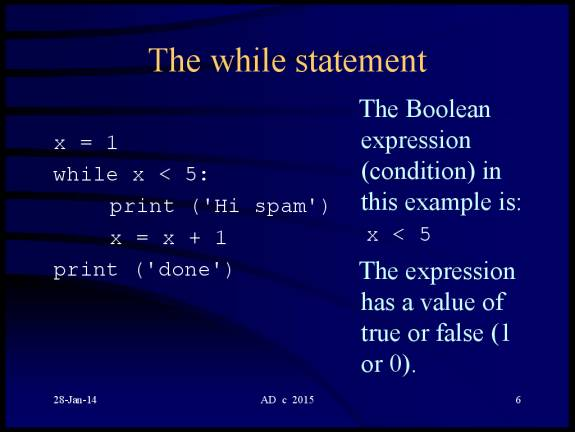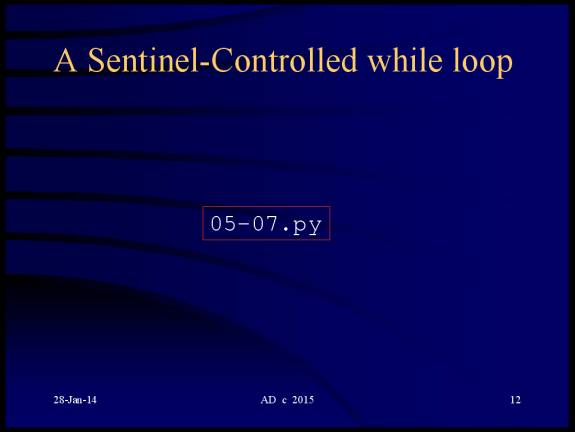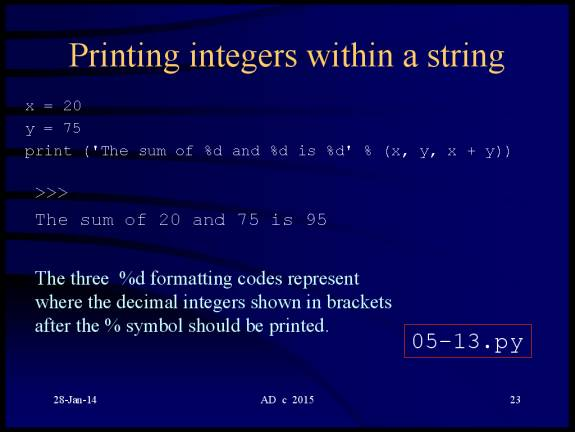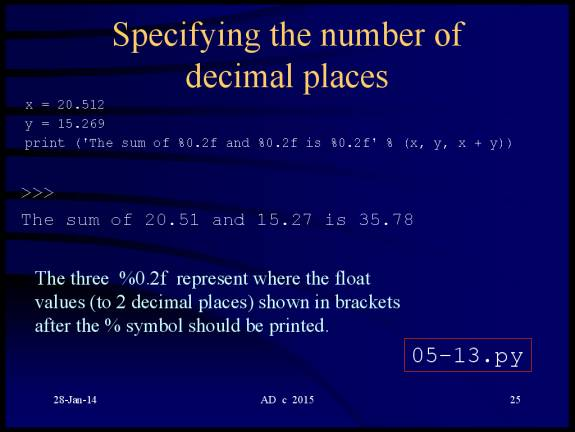```

```
```

```

# The while statement

Like the if  statement, the while  statement makes use of a  Boolean expression...

## print ('done')

The Boolean expression (condition) in this example is:

x < 5

The expression has a value of true or false (1 or 0).

If the condition is true,

the indented statements are executed,

otherwise, the statements are skipped.## http://www.annedawson.net/python3programs.html# The break statement# The continue statement` `

# A Sentinel-controlled while loop` `

## http://www.annedawson.net/python3programs.html

`#  File:       05-07.py `
`#  Purpose:    Example: 'sentinel-controlled' while loop`
`#              Calculates average score of a class`
`#  Programmer: Anne Dawson`
`#  Course:     CSCI120A`
`#  Date:       Tuesday 5th October 2004, 6:31 PT`
` `
` `
`# initialization phase`
`totalScore = 0     # sum of scores`
`numberScores = 0   # number of scores entered`
` `
`# processing phase`
`score = input( "Enter score, (Enter -9 to end): " )   # get one score`
`score = int( score )   # convert string to an integer`
` `
`while score != -9: # -9 is used as a sentinel ( a lookout or sentry value )`
`    totalScore = totalScore + score`
`    numberScores = numberScores + 1`
`    score = input( "Enter score, (Enter -9 to end): " )  `
`    score = int( score )`
`   `
`# termination phase`
`if numberScores != 0: # division by zero would be a run-time error`
`   average = float( totalScore ) / numberScores`
`   print ("Class average is", average)`
`else:`
`   print ("No scores were entered")`
` `
` `
```
```
```

```
```

```

# Counter-controlled repetition with the for loop## http://www.annedawson.net/python3programs.html# Using continue with the for loop

## print (c)# Using break with the for loop

## print (c)```

```

# Printing strings and numbers

## print ('Total is: ', d, 'dollars and', c, ' cents')#depending on the context.

In the following example we see the % operator

being used to specify how a string should be printed

(i.e. string formatting).## http://www.annedawson.net/python3programs.html## http://www.annedawson.net/python3programs.html## http://www.annedawson.net/python3programs.html

```
```
```
```

Example program 05-14.py illustrates

how to repeat a program at the user's request:

#  File:       05-14.py

#  Purpose:    Example: how to repeat a program at the user's request

#  Programmer: Anne Dawson

#  Course:     CSCI120A, CSCI165

#  Date:       Thursday 19th October 2006, 7:58 PT

print ("This is the start of the program")

print ("This is a statement from within the while loop")

print ("This is another statement from within the while loop")

answer = input("Do you want to run this program again? y/n")

print ("Goodbye!")

## http://www.annedawson.net/python3programs.htmlExample program 05-15.py - 05-18.py

illustrate how to use loops inside loops:

`#  File:       05-17.py `
`#  Purpose:    Example: how to use a loop within a loop`
`#              a nested for loop`
`#  Programmer: Anne Dawson`
`#  Course:     CSCI120A, CSCI165`
`#  Date:       Wednesday 27th June 2007, 9:45 PT`
` `
`print("This is the start of the program")`
` `
`for i in range(1,6):`
`    for j in range(1,6):`
`        print ("i: " + str(i) + " j: " + str(j) )`
`    print()        `
`'''`
`Notice that with a loop repeating 5 times,`
`***within*** a loop that repeats 5 times`
`means that you can control 25 processes.`
`'''`

## http://www.annedawson.net/python3programs.html

The output after the program runs:

This is the start of the program

i: 1 j: 1

i: 1 j: 2

i: 1 j: 3

i: 1 j: 4

i: 1 j: 5

i: 2 j: 1

i: 2 j: 2

i: 2 j: 3

i: 2 j: 4

i: 2 j: 5

i: 3 j: 1

i: 3 j: 2

i: 3 j: 3

i: 3 j: 4

i: 3 j: 5

i: 4 j: 1

i: 4 j: 2

i: 4 j: 3

i: 4 j: 4

i: 4 j: 5

i: 5 j: 1

i: 5 j: 2

i: 5 j: 3

i: 5 j: 4

i: 5 j: 5